Journal of Integer Sequences, Vol. 21 (2018), Article 18.6.5

## Lucasnomial Fuss-Catalan Numbers and Related Divisibility Questions

### Christian Ballot Département de Mathématiques et Informatique Université de Caen-Normandie France

Abstract:

For all integers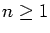we define the generalized Lucasnomial Fuss-Catalan numbers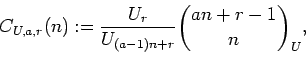and prove their integrality. Here U is a fundamental Lucas sequence,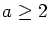and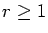are integers, and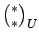denotes a Lucasnomial coefficient. If U = I, where In = n, then the CI,a,r(n) are the usual generalized Fuss-Catalan numbers. With the assumption that U is regular, we show that U(a-1)n+k divides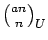for allbut a set of asymptotic density 0 if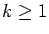, but only for a small set if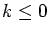. This small set is finite when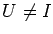and at most of upper asymptotic density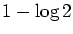when U = I. We also determine all triples (U,a,k), where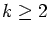, for which the exceptional set of density 0 is actually finite, and in fact empty.

Full version:  pdf,    dvi,    ps,    latex

(Concerned with sequences A001764 A003150 A014847 A107920.)

Received March 6 2018; revised version received June 6 2018. Published in Journal of Integer Sequences, August 22 2018.